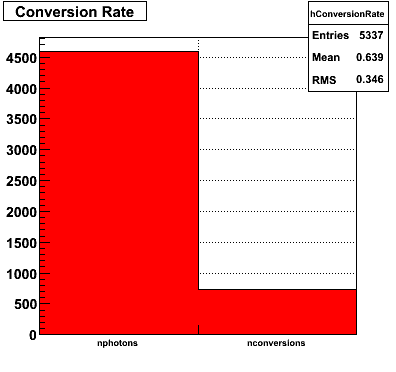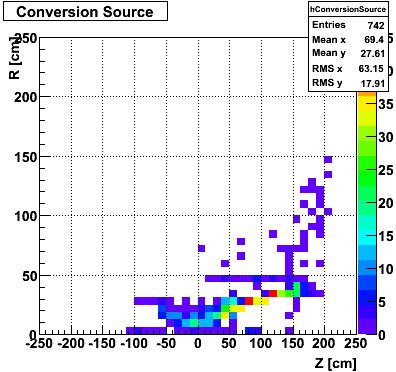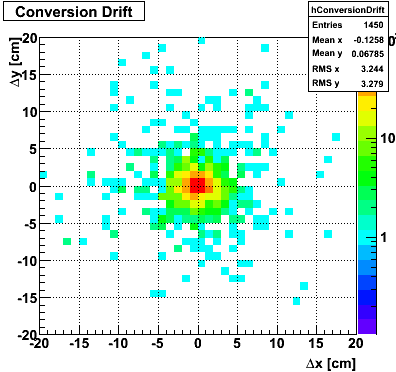# Data-Driven Shower Shapes

## Gamma Conversion before the Endcap

The plots below show the conversion process before the Endcap. I look at prompt photons heading towards the Endcap from a MC gamma-jet sample with a partonic pT of 9-11 GeV. I identify those photons that convert using the GEANT record. The top left plot shows the total number of direct photons and those that convert. I register a 16% conversion rate. This is consistent with Jason's 2006 SVT review. The top right plot shows the source of conversion, where most of the conversions emanate from the SVT support cone, also consistent with Jason's study. The bottom left plot shows the separation in the SMD between the projected location of the photon and the location of the electron/positron from conversion.## Shower shapes comparison

This

PDF

file shows several shower shapes in a single plot for comparison:

• MC - Monte Carlo shower shape from the 9-11 GeV pT gamma-jet Pythia sample
• DD - Data-driven Monte Carlo shower shape (Each final state photon shower shape is replaced with a corresponding shower shape from data in the same sector configuration, energy, preshower, and U/V-plane bin).
• Standard MC - Monte Carlo shower shape parametrized by Hal (also from the 9-11 GeV pT gamma-jet Pythia sample)
• Will - Data shower shape derived from photons from eta decays by Will using a modified version of Weihong/Jason meson pi0 finder
• Pibero - Data shower shape derived from photons from eta decays by Pibero using a crude eta finder

## Shower Shapes Sorted by SMD Plane, Sector Configuration, Energy and Preshower

These Shower Shapes are binned by:

1. SMD plane (U and V)
2. Sector configuration with the formula sector%3 where sector=1..12, so 3 different bins. More details can be found at the EEMC Web site under the Geometry link.
3. Energy of the photon (E < 8 GeV and E > 8 GeV)
4. Preshower energy `(pre1==0&amp;&amp;pre2==0)` and `(pre1&gt;0||pre2&gt;0)`

They are then fitted with a triple-Gaussian of the form:

`*(*exp(-0.5*((x-)/)**2)/(sqrt(2*pi)*)+*exp(-0.5*((x-)/)**2)/(sqrt(2*pi)*)+(1--)*exp(-0.5*((x-)/)**2)/(sqrt(2*pi)*))`

## Comparison of Sided Residuals for Monte Carlo (MC) and Data-Driven (DD) Shower Shapes

All fits to MC are with reference to the old Monte carlo fit function:

`*(0.69*exp(-0.5*((x-)/0.87)**2)/(sqrt(2*pi)*0.87)+0.31*exp(-0.5*((x-)/3.3)**2)/(sqrt(2*pi)*3.3))`

All fits to the data are with reference to a single

Shower Shape

. The fit function is:

`*(*exp(-0.5*((x-)/)**2)/(sqrt(2*pi)*)+*exp(-0.5*((x-)/)**2)/(sqrt(2*pi)*)+(1--)*exp(-0.5*((x-)/)**2)/(sqrt(2*pi)*))`

## Comparison of Sided Raw Tails for Monte Carlo (MC) and Data-Driven (DD) Shower Shapes

Pibero Djawotho
Last updated Tue Apr 8 17:29:40 EDT 2008